# What are Square Numbers?

What are Square Numbers?The first twelve square numbers are: 1, 4, 9, 16, 25, 36, 49, 64, 81, 100, 121 and 144.• To find a square number, we multiply a whole number by itself.
• When we multiply a number by itself we get the area of a square.
• ‘Squaring’ a number means to multiply the number by itself.
• We write a small ‘2’ above our number to tell us to square it.
• For example, 5 squared is written as 52, which means 5 × 5, which equals 25.• To find the first five square numbers, we multiply each number by itself.
• 1 x 1 = 1.
• 2 x 2 = 4.
• 3 x 3 = 9.
• 4 x 4 = 16.
• 5 x 5 = 25.
• The first five square numbers are 1, 4, 9, 16 and 25.# Square Numbers

## What are Square Numbers?

A square number is the result when any number is multiplied by itself. For example 3 × 3 = 9 and so, 9 is a square number. The numbers of 1, 4, 9, 16, 25, 36, 49, 64, 81, 100, 121 and 144 are all square numbers.

A square number is formed when any whole number multiplied by itself.

Here is a list of the first 12 square numbers from one squared up to twelve squared.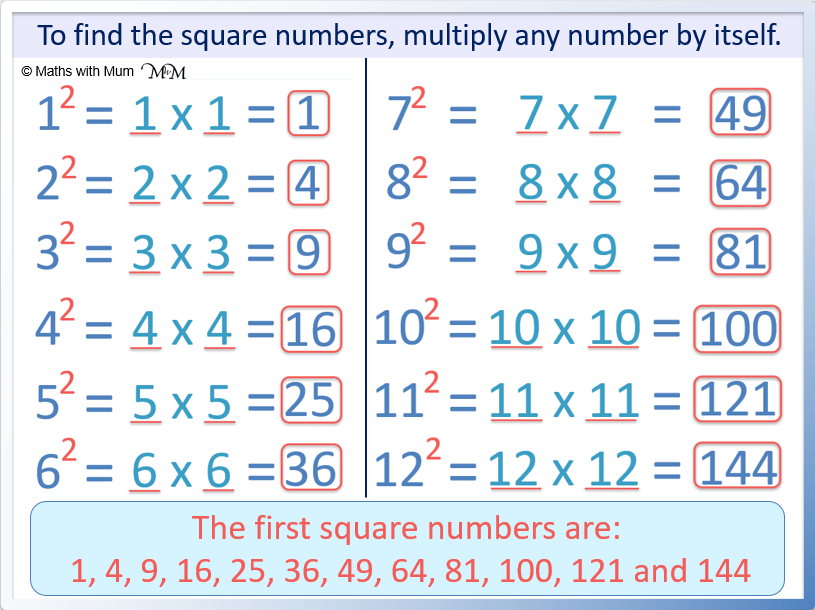‘Squaring a number’ means to multiply the number by itself.

For example, 5 squared means 5 × 5. 5 squared equals 25.

All numbers can be squared, no matter how large. For example, 999 × 999 = 998001.

## List of Square Numbers From 1 to 100

The square numbers from 1 to 100 are 1, 4, 9, 16, 25, 36, 49, 81 and 100. 100 is the tenth square number because it is 10 × 10.Here is a list of the first 12 square numbers:

Number Calculation Square Number
12 1 × 1 = 1
22 2 × 2 = 4
32 3 × 3 = 9
42 4 × 4 = 16
52 5 × 5 = 25
62 6 × 6 = 36
72 7 × 7 = 49
82 8 × 8 = 64
92 9 × 9 = 81
102 10 × 10 = 100
112 11 × 11 = 121
122 12 × 12 = 144

## List of the First 100 Square Numbers

The first 100 square numbers are: 1, 4, 9, 16, 25, 36, 49, 64, 81, 100, 121, 144, 169, 196, 225, 256, 289, 324, 361, 400, 441, 484, 529, 576, 625, 676, 729, 784, 841, 900, 961, 1024, 1089, 1156, 1225, 1296, 1369, 1444, 1521, 1600, 1681, 1764, 1849, 1936, 2025, 2116, 2209, 2304, 2401, 2500, 2601, 2704, 2809, 2916, 3025, 3136, 3249, 3364, 3481, 3600, 3721, 3844, 3969, 4096, 4225, 4356, 4489, 4624, 4761, 4900, 5041, 5184, 5329, 5476, 5625, 5776, 5929, 6084, 6241, 6400, 6561, 6724, 6889, 7056, 7225, 7396, 7569, 7744, 7921, 8100, 8281, 8464, 8649, 8836, 9025, 9216, 9409, 9604, 9801 and 10000.

The first 100 square numbers are shown in the table below.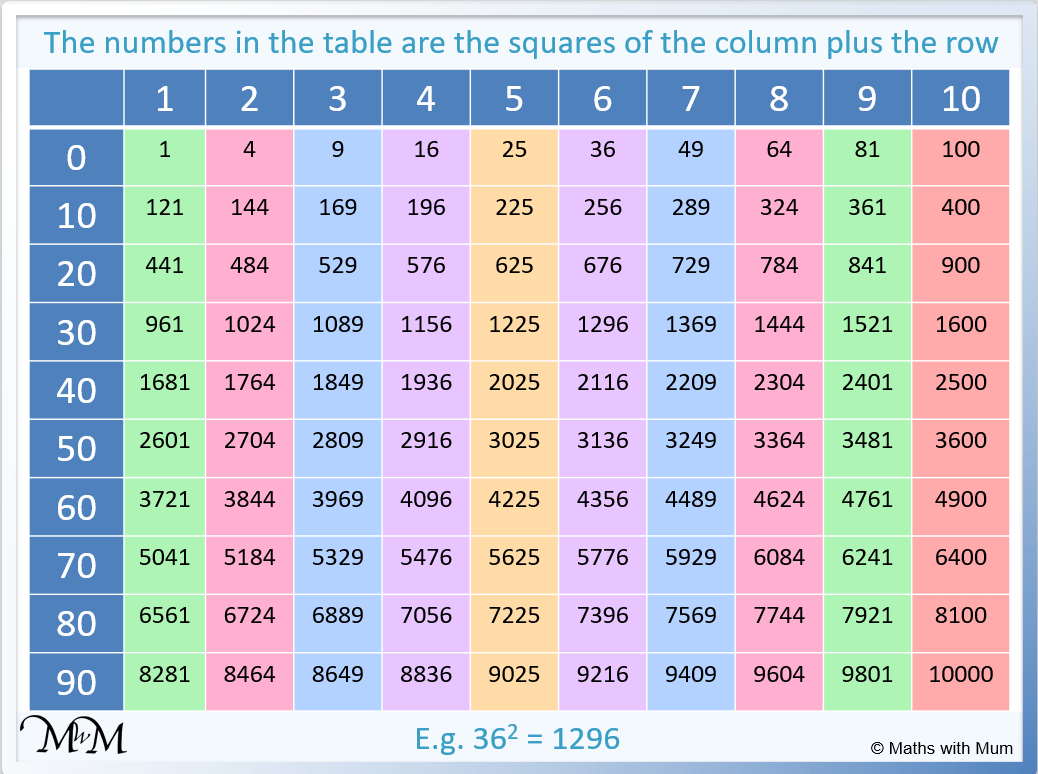To use the square number chart above, simply add the numbers at the end of each column and row to find the number you wish to square. The square of this number is where the column and row meet.

For example to find 36 squared, look in the 30 row and the 6 column. We can see that these meet at the number 1296. Therefore 362 = 1296.

Another example of using the square number chart is 912. We look in the 90 row and the 1 column. 912 = 8281.

## Square Numbers Chart

Here is a number chart showing the square numbers. The square numbers form a diagonal pattern on a multiplication chart.Here are the square numbers shown on a number grid to 100.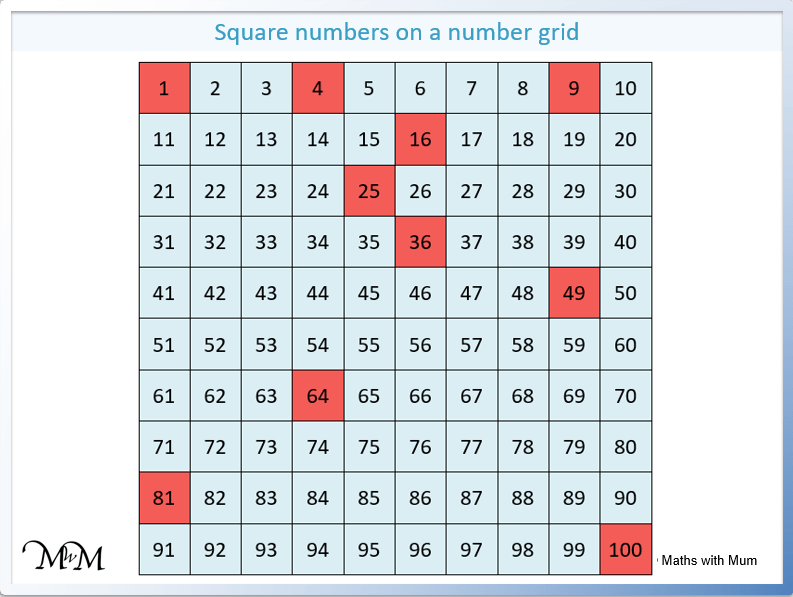We can see that the square numbers get more and more spread out because the difference from one square number to the next increases as the square numbers get larger.

## What is a Perfect Square?

A perfect square is another name for a square number. A perfect square is any number that can be formed by multiplying a whole number by itself. For example, 25 is a perfect square because 25 = 5 × 5 and 15 is not a perfect square because it can only be written as 1 × 15 or 3 × 5.

To test if a number is a perfect square, find its square root. If the square root is a whole number, the number is a perfect square. If the square root is a decimal number, then the number is not a perfect square.

It is impossible to have a decimal square number because a whole number multiplied by a whole number will always make another whole number.

The name perfect square is used to confirm to the person reading that we are specifically talking about squaring a whole number, rather than squaring any other number such as a decimal.

## Properties of Square Numbers

• Square numbers always end in 0, 1, 4, 5, 6 or 9.
• Square numbers are always positive.
• Square numbers always have a whole number as their square root.
• Square numbers never end in an odd number of zeros.
• Square numbers are either in the 4 times table or are one away from a number in the 4 times table.

Here is a chart showing the square numbers in order, arranged by their last digit. We can see that the square numbers only end in 0, 1, 4, 5, 6 or 9.A square number is always positive. This is because a positive number multiplied by a positive number is positive and a negative number multiplied by a negative number is also positive.

For example, if we try to square -3, we get -3 × -3. A negative times a negative is always positive as two negative numbers multiplied together cancel out. -3 × -3 = +9.

Whenever we square a negative number, we end up with a positive.

Adding up the odd numbers from 1 always results in a square number. Adding the first n odd numbers equals the nth square number. For example, to find the 5th square number, we add the first 5 odd numbers. 1 + 3 + 5 + 7 + 9 = 25, which is 5 × 5.Adding odd numbers from 1 onwards always produces a square number.

Here is a table showing square numbers as a sum of odd numbers.

n Sum of n odd numbers nth square number
1 1 = 1 = 1 × 1
2 1 + 3 = 4 = 2 × 2
3 1 + 3 + 5 = 9 = 3 × 3
4 1 + 3 + 5 + 7 = 16 = 4 × 4
5 1 + 3 + 5 + 7 + 9 = 25 = 5 × 5
6 1 + 3 + 5 + 7 + 9 + 11 = 36 = 6 × 6
7 1 + 3 + 5 + 7 + 9 + 11 + 13 = 49 = 7 × 7
8 1 + 3 + 5 + 7 + 9 + 11 + 13 + 15 = 64 = 8 × 8
9 1 + 3 + 5 + 7 + 9 + 11 + 13 + 15 + 17 = 81 = 9 × 9
10 1 + 3 + 5 + 7 + 9 + 11 + 13 + 15 + 17 + 19 = 100 = 10 × 10
11 1 + 3 + 5 + 7 + 9 + 11 + 13 + 15 + 17 + 19 + 21 = 121 = 11 × 11
12 1 + 3 + 5 + 7 + 9 + 11 + 13 + 15 + 17 + 19 + 21 + 23 = 144 = 12 × 12

Each square number is always an odd number away from the previous square number. To get from one square number to the next, add the next odd number.

The square numbers are 1, 4, 9, 16, 25, 36, 49, 64, 81, 100, 121, 144 and so on.

The difference from one square number to the next is 3 then 5, then 7 and so on.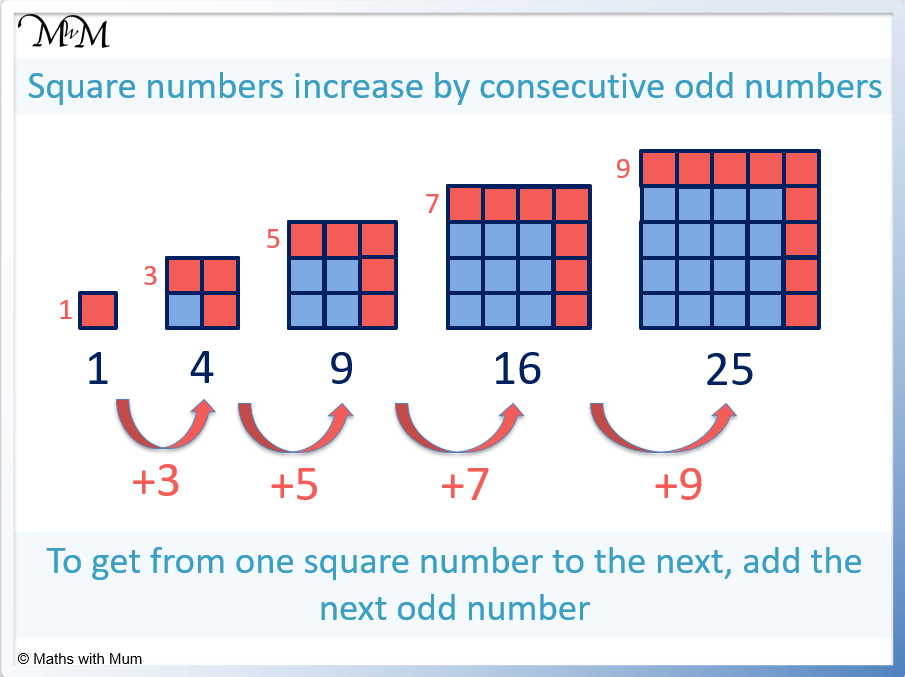Here is a table showing how any square number can be found by adding an odd number on to the previous square number.

Square Number Odd Number To Add Next Square Number
0 + 1 = 1
1 + 3 = 4
4 + 5 = 9
9 + 7 = 16
16 + 9 = 25
25 + 11 = 36
36 + 13 = 49
49 + 15 = 64
64 + 17 = 81
81 + 19 = 100
100 + 21 = 121
121 + 23 = 144

## Square Numbers That Are Also Cube Numbers

Numbers that are both square and cube are the powers of 6. These numbers are: 1, 64, 729, 4096, 15625 and so on.

## Square Numbers That Are Odd

All odd square numbers are one more than a multiple of 4. The odd square numbers are 1, 9, 25, 49, 81, 121, 169 and so on. The odd square numbers are always formed by multiplying an odd number by itself.

## Square Numbers That Are Even

All even square numbers are multiples of 4. The even square numbers are 4, 16, 36, 64, 100 and so on. This is because any even number can be written as 2n and (2n)2 = 4n2. This means that the even square numbers can be formed by multiplying the original list of square numbers by 4.

## Square Numbers Cannot Be Prime

Prime numbers only have two factors, one and themselves. Square numbers always have their square root as an extra factor and so, cannot be prime. For example, 9 has the factors of 1 and 9 but it also has its square root 3 as a factor.

## Why Are Square Numbers Called Square Numbers?

Square numbers are called this because they form the area of a square. Squares have sides that are the same length, so finding the area of a square is found by multiplying one side length by itself. ‘Squaring’ one of the sides of a square tells us its area.

Here are some of the first square numbers shown as squares.

Square Number Example 1: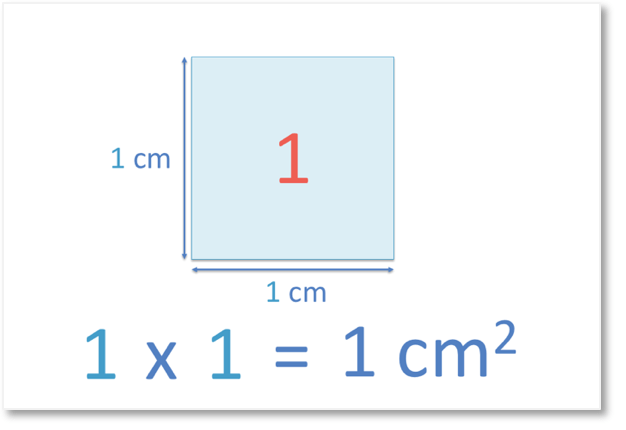A side length of this square is 1 cm.

To find the area of this square, we multiply 1 by itself.

1 x 1 = 1

The area of the square is 1 cm2.

Therefore, 1 is a square number.

One is the first square number.

When teaching square numbers, be aware of a common mistake of students thinking that one squared is two. Often students will see 12 and do one times two rather than one times itself. This mistake occurs frequently with this example of calculating one squared but it can occur with all square number calculations and is worth reinforcing.

Square Number Example 2:A side length of this square is 2 cm.

To find the area of this square, we multiply 2 by itself.

2 x 2 = 4

The area of the square is 4 cm2.

Therefore, 4 is a square number.

Four is the second square number.

Square Number Example 3:A side length of this square is 3 cm.

To find the area of this square, we multiply 3 by itself.

3 x 3 = 9

The area of the square is 9 cm2.

Therefore, 9 is a square number.

Nine is the third square number.

Square Number Example 4: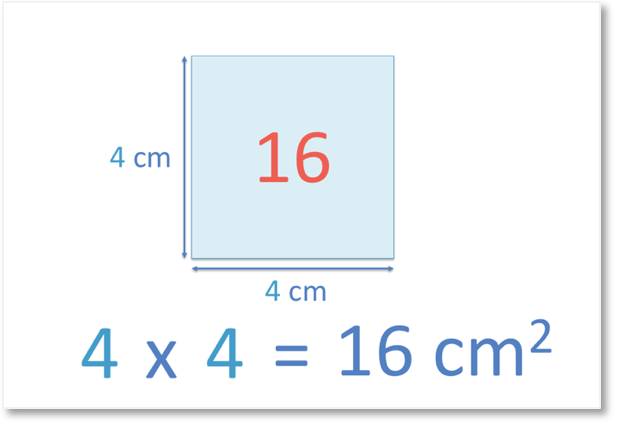A side length of this square is 4 cm.

To find the area of this square, we multiply 4 by itself.

4 x 4 = 16

The area of the square is 16 cm2.

Therefore, 16 is a square number.

Four squared is 16.Now try our lesson on What are Perfect Square Roots? where we learn what square roots are and how we find them.

error: Content is protected !!